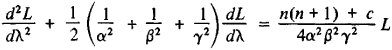Lamé Functions

(redirected from Lame Functions)

Lamé functions

[lä′mā ‚fəŋk·shənz]
(mathematics)
Functions that arise when Laplace's equation is separated in ellipsoidal coordinates.

Lamé Functions

functions used in studying physical phenomena (distribution of heat, the motion of a fluid) in regions bounded by the surface of an ellipsoid. Lamé functions L(λ) are the simplest solutions of the Lamé differential equationwhere a2 = a2+ λ, β2 = b2+ λ, y2 = c2+ λ, n is an integer, and a, b, and c are the semiaxes of the ellipsoid inside (or outside) of which the physical phenomenon is to be studied. Lamé functions, introduced by G. Lamé in 1839, find many applications in various problems of mathematical physics and mechanics.

REFERENCES

Smirnov, V. I. Kurs vysshei matematiki, 6th ed., vol. 3, part 2. Moscow-Leningrad, 1957.
Whittaker, E. T., and G. N. Watson. Kurs sovremennogo analiza, 2nd ed., part 2. Moscow, 1963. (Translated from English.)
Mentioned in ?
References in periodicals archive ?
Cook and Fowler  sought to explore solutions of the temporally independent Schroedinger equation for the hydrogen atom in spheroconical coordinates in terms of Lame functions of the first and second kind, but produced neither an explicit formula for a spheroconical amplitude function nor a plot thereof.

Site: Follow: Share:
Open / Close